# Texas Go Math Grade 3 Unit 6 Answer Key Personal Financial Literacy

Refer to our Texas Go Math Grade 3 Answer Key Pdf to score good marks in the exams. Test yourself by practicing the problems from Texas Go Math Grade 3 Unit 6 Answer Key Personal Financial Literacy.

## Texas Go Math Grade 3 Unit 6 Answer Key Personal Financial Literacy

Show What You Know

Check your understanding of important skills

Practice Multiplication Facts: Find the product.

Question 1.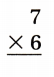Explanation:
The product of 7 and 6 is 42

Question 2.
4 × 8 = _________
Explanation:
The product of 4 and 8  is 32

10 × 5 = __________
Explanation:
The product of 10 and 5 is 50

Question 4.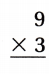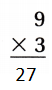Explanation:
The product of 9 and 3 is 27

Question 5.
_______ = 0 × 6
Explanation:
The product of 0 and 6 is 0
When some thing is multiplied with 0 the answer is 0

Record 2-Digit Addition and Subtraction: Find the sum or difference.

Question 6.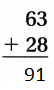Explanation:
The sum of
Two digit addition by using borrow

Question 7.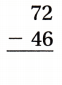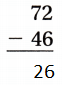Explanation:
The difference of
Two digit subtraction by using borrow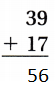Explanation:
The sum of
Two digit addition by using borrow

Question 9.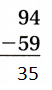Explanation:
The difference of
Two digit subtraction by using borrow

Question 10.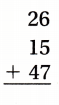Explanation:
The sum of
Two digit addition by using borrow

3-Digit Addition and Subtraction with Regrouping: Find the sum or difference.

Question 11.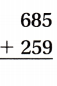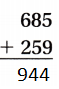Explanation:
The sum of
Three digit addition by using borrow

Question 12.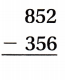Explanation:
The difference of
Three digit subtraction by using borrow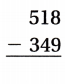Explanation:
The difference of
Three digit subtraction by using borrow

Question 14.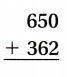Explanation:
The sum of
Three digit addition by using borrow

Question 15.Explanation:
The difference of
Three digit subtraction by using borrow

Question 16.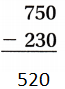Explanation:
The difference of
Three digit subtraction by using borrow

Question 17.Explanation:
The sum of
Three digit addition by using borrow

Question 18.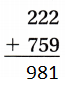Explanation:
The sum of
Three digit addition by using borrow

Vocabulary Builder

Visualize It ……………..

Use the checked words to complete the word map.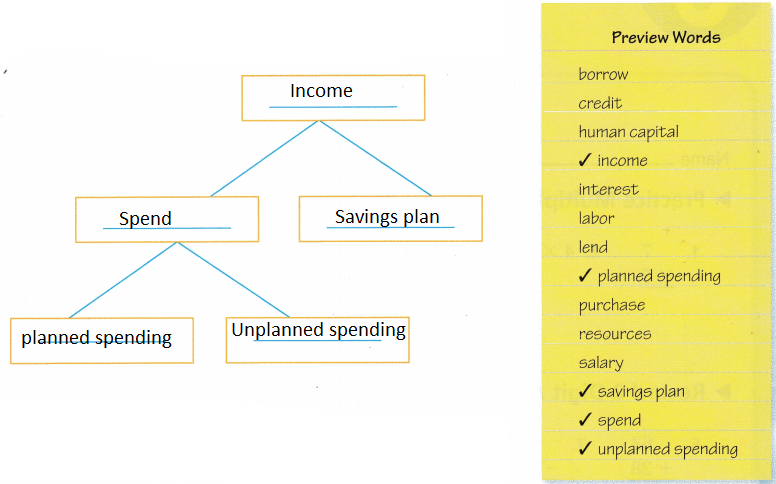Explanation:
The word map is completed by using preview words
When we get income we have 2 options either to save or spend
in spending we have 2 options planned and unplanned spending’s.

Understand Vocabulary

Complete the sentences by using the preview words.

Question 1.
You ___________ something when you obtain it by paying money for an item or a service.
Explanation:
The action of buying something. A thing that has been bought

Question 2.
Money earned is called _____________.
income is the money we use to fund our day-to-day expenditures. The income may be received in the form of wages, salary, freelance payments, or the receipts of a small business. Investments, pensions, Social Security, and other government benefits programs may also be sources of income.

You ___________ money when another person gives you money that you will need to pay back.
Explanation:
Take and use (something belonging to someone else) with the intention of returning it.
“he had borrowed a car from one of his colleagues”

Question 4.
When you _____________ money, you give it to someone else in exchange for something.
Explanation:
Give (money) to pay for goods, services, or so as to benefit someone or something.

Question 5.
Explanation:
Savings plans are financial products designed to enable disciplined savings while delivering steady returns that help you achieve your financial goals. As they are primarily a life insurance product, these plans also ensure the financial security of your loved ones in case something happens to you.

Question 6.
You _____________  money to someone else by giving money that needs to he paid back.
Explanation:
Grant to (someone) the use of (something) on the understanding that it will be returned.
“Stewart asked me to lend him my car”

Vocabulary

On the way home from school, Jan inc spends 25 cents to buy a banana. She gives the grocer a \$1 bill. The change is the amount of money she gets back. How much change should she get back?

Look at the coins in each box. Would Jan me get the correct change if the grocer gave her these coins?

Write exactly correct, not enough change, or too much change.

Question 1.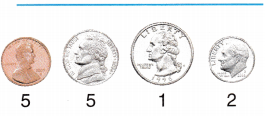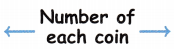Question 2.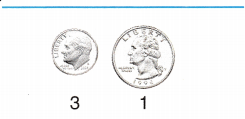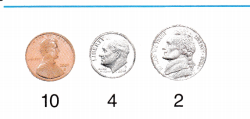Question 4.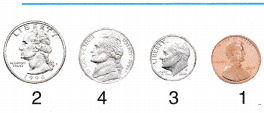Writing Write about what you might spend money on.

Reading Look for this book in the library. Jelly Beans for Sale, by Bruce McMillanAnswer: Mostly we spend money on shopping

Count It Up!
Object of the Game Practice counting money amounts.

Materials

• set of 20 index cards with random money amounts up to one dollar
• play money (5 half-dollars, 5 quarters, 10 dimes, 15 nickels, and 50 or more pennies)
• clock or timer

Set Up
Divide students into pairs or small groups. Each pair or small group receives the same amount of play money.
Number of Players 2 teams of 2-4 players

How to Play

1. Place index cards facedown. Team 1 chooses an index card and announces the target amount.2. Team 1 gets 3 minutes to make as many different combinations of coins that equal the target amount as possible. Team 1 records the number of different combinations made.

3. Team 2 chooses a card and follows the directions in Step 2. The team to make the most correct different combinations of coins scores 1 point.

4. Continue until all the index cards are used. The team with the greatest number of points wins.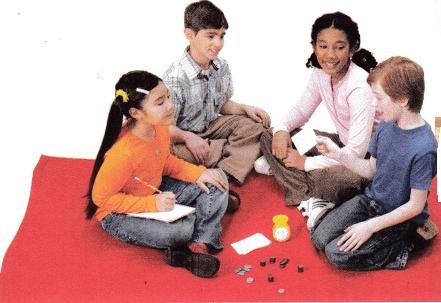Answer: This is a mind game with the coins
Through this type of games, children get to know the coins and their values

Scroll to Top